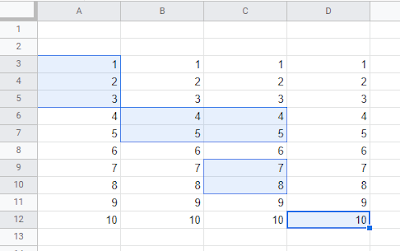## Tuesday 24 May 2022

### Get all selected Ranges in a Google Sheet

The following Google Apps Script was from some learning around multiple ranges being selected/highlighted by a user in a Google Sheet. I have always worked with individual cells or continuous ranges within Sheets, but during the development of my Archive Sheet data Add-on I wanted to enhance it by allowing more rows to be acted upon in one go.

I practiced getting a few values after looping through each selected range:

• A1 Notation (e.g. A2:C5),
• Cell values,
• Starting row,
• End row,
• Number of rows (calculated from the above),
• Starting column,
• End column,
• Number of columns (calculated from the above).Selected ranges in a Google Sheet

### The Code

Here are some highlights of the Apps Script code:

When getting all selected/highlighted ranges we are returned an array:

var ranges = ss.getActiveRangeList().getRanges();

Which means we need to loop through each item so that we can get some of the specific details listed above:

for (var i = 0; i < ranges.length; i++) {

// get first range of cells as a variable so easier to use
var individualRange = ranges[i];

// get A1 Notation for range of cells
var rangeNotation = individualRange.getA1Notation();

// get values of cells in range
var rangeCellValues = individualRange.getValues();

// get starting Row of range
var rangeStartRow = individualRange.getRow();

// get last Row of range
var rangeLastRow = individualRange.getLastRow();

// calculate the number of Rows in the range
var noOfRows = (rangeLastRow - rangeStartRow) + 1;

// get starting Column of range
var rangeStartCol = individualRange.getColumn();

// get last Column of range
var rangeLastCol = individualRange.getLastColumn();

// calculate the number of Columns in the range
var noOfCols = (rangeLastCol - rangeStartCol) + 1;

}